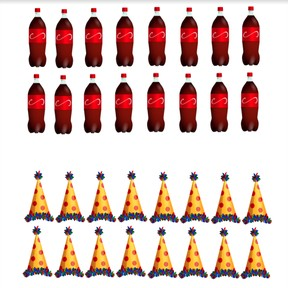Recognizing equal quantities to 20

# Recognizing equal quantities to 20

Recognizing equal quantities to 208,000 schools use Gynzy92,000 teachers use Gynzy1,600,000 students use Gynzy

## General

Students recognize equal quantities to 20. They learn this by comparing different images and objects with each other.

1.NBT.B.3

## Relevance

It is useful to know if you have more, less or equal quantities of things. An example would be if you want to know if you have enough chairs set out for a party.

## Introduction

Count all the students in the classroom. Group them into boys/girls. Ask the student to count books, chairs, pens, or other objects to 20 within the classroom in pairs.

## Development

Show the image with bottles and party hats. Are there equal quantities? How do you know? Count the first group out loud and then count the second group out loud. Are the quantities the same? If they are, they are equal quantities. Practice this by comparing the images of cookies and plants to show the difference. Check that students recognize equal quantities by doing a short practice. Ask the students to hold up a green pencil if they believe that the quantities shown are equal, and to hold up a red pencil if they are not equal quantities. You can also give cards or any other device to check that they understand.

To check that students understand what equal quantities are you can ask the following questions:
What does equal quantities mean?
Do you count equal quantities of candles on the cakes?
How do you know if there are equal quantities?

## Guided Practice

The students practice by comparing different images with quantities to 20 with dots and are asked to indicate if they are equal quantities or not.

## Closing

Check that students understand equal quantities by asking them to drag an equal quantities of presents to the right box. Ask the students how they can check their answer. They can count both quantities again to check that they are the same.

## Teaching Tip

Students who have difficulty should be encouraged to practice counting to 20 and comparing quantities to 20 with each other. Students who are ready for more challenge can be encouraged to compare numbers to 20 without images.

## Instruction materials

Red and green pencils.

### The online teaching platform for interactive whiteboards and displays in schools

• Save time building lessons

• Manage the classroom more efficiently

• Increase student engagement